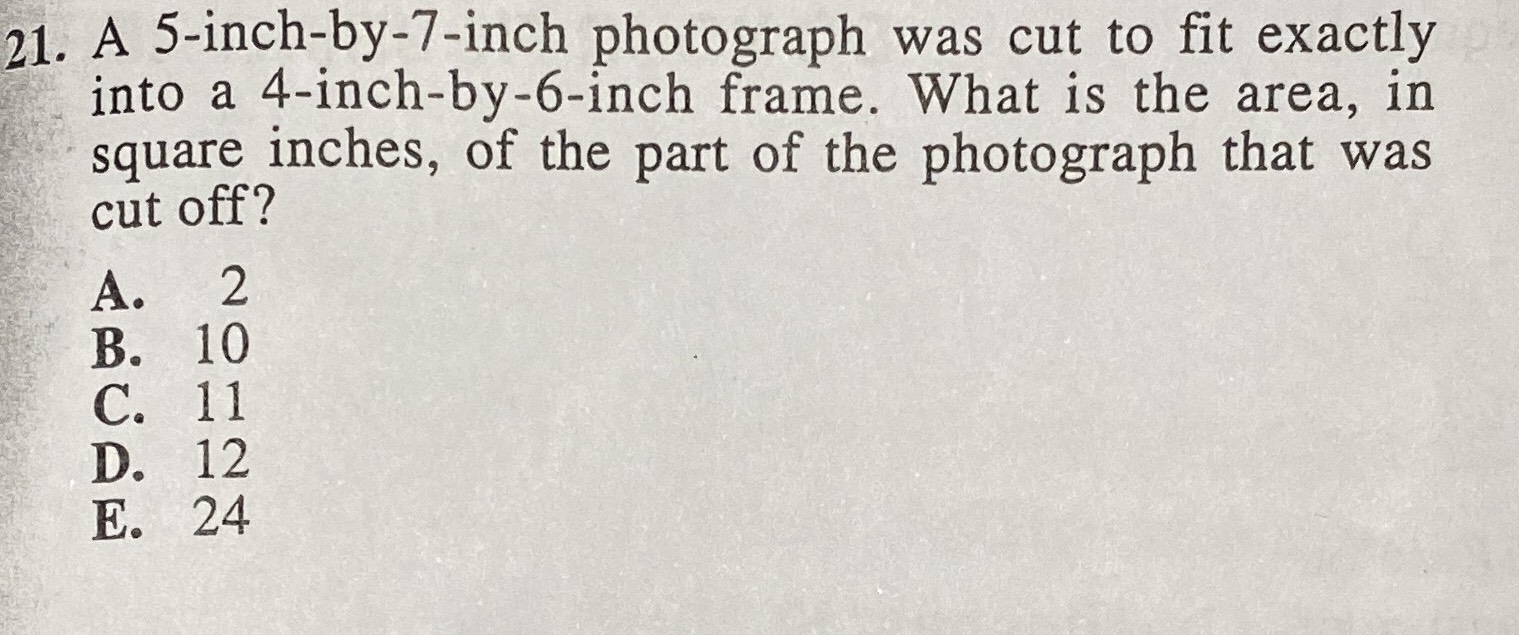### ¿Todavía tienes preguntas de matemáticas?

Pregunte a nuestros tutores expertos
Algebra
Pregunta21. A $$5$$ -inch-by-7-inch photograph was cut to fit exactly into a $$4$$ -inch-by-6-inch frame. What is the area, in square inches, of the part of the photograph that was cut off? A. $$2$$ B. $$10$$ C. $$11$$ D. $$12$$ E. $$24$$